• Introduction
• What is in this manual
• What is Caspoc
• User interface
• Introduction
• Starting
• Simulation
• Editing
• Viewing and printing
• Getting Started
• Basic editing
• Simulation in the time domain
• Basic User Interface Topics
• Editing
• Simulation
• Viewing
• Library
• Reports
• Project management
• Circuit and Block Diagram Components
• Introduction
• Cscript and user defined functions
• Component parameters
• Modeling Topics
• Introduction
• Power Electronics
• Semiconductors
• Electrical Machines
• Electrical drives
• Power Systems
• Mechanical Systems
• Thermal Systems
• Magnetic Circuits
• Green Energy
• Coupling to FEM
• Experimenter
• Analog hardware description language
• Embedded C code Export
• Coupling to Spice
• Small Signal Analysis
• Matlab coupling
• Tips and tricks
• Appendices

## Create your first time domain system simulation.

In this tutorial we will create a voltage source where the voltage is defined in a block diagram. In the block diagram we model a sinusoidal wave form. This sinusoidal wave form is the input for the controlled votlage source. Create the circuit as shown below. Select the controlled voltage source B from Components/Circuit/Controlled Sources and the resistor and inductor from Components/Circuit/RLC
The block TIME and SIGNAL are selcted from Components/Blocks/Source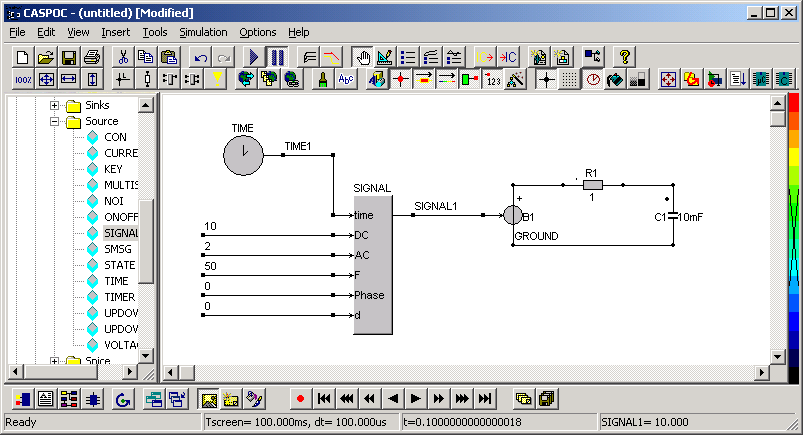The block SIGNAL is used for creating the sinusoidal wave form. Open the block SIGNAL by clicking it with the right mouse button. The third parameter indicates the type of the signal. There are various wave forms possible such as sinusoidal, square wave, triangular, etc. Change the third parameter into 3 to select a sinusoidal wave form.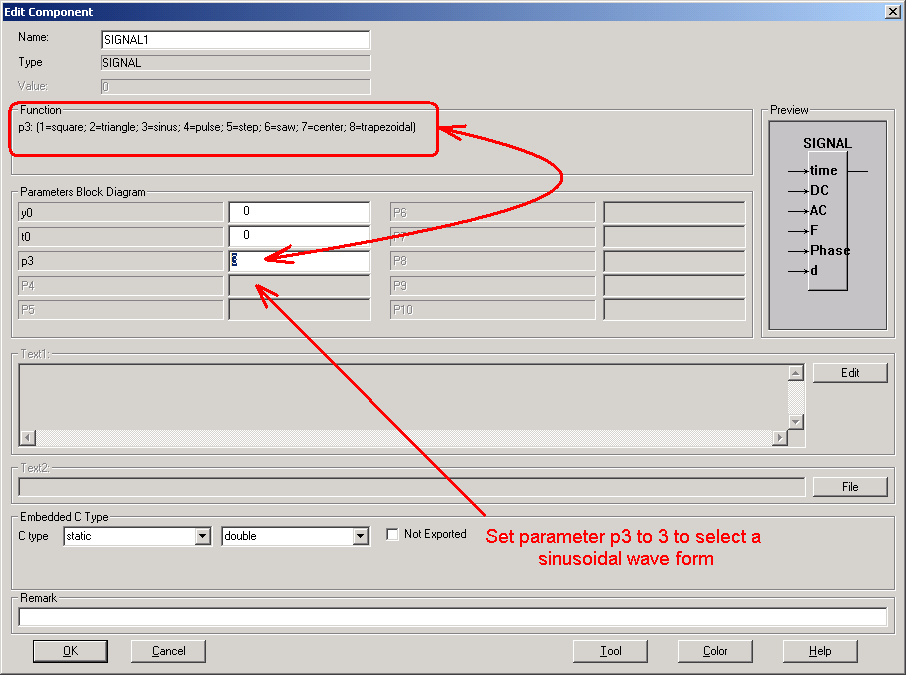The resistor has a value of 1 ohm and the capacitor hasa value of 10mF. For the block signal we define the parameters at the input of the block. The first input is connected to the block TIME, being the simulation time. The second input is the DC value of the wave form. The third input is the AC value of the wave form. The fourth and fifth input are the frequency and phase of the signal. The last input of the block SIGNAL defines the duty cycle for square wave forms. Since we a re modeling a sinusoidal wave form, this parameter is not used and set to zero.
We want to display the output voltage, capacitor current and the signal from the block SIGNAL in one scope. Select a scope from the button bar at the bottom of your window.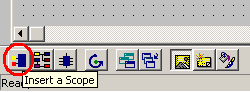Enlarge the scope to create more inputs. We want to display the output voltage, capacitor current and the output from the block SIGNAL, so three inputs are required. Click and drag the bottom-right corner of the scope with the left mouse button clicked. During the resizing of the scope, the number of inputs will be shown.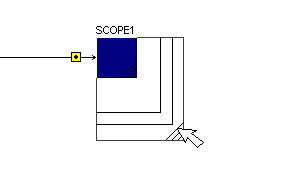If the input from a scope is over a node, its input connection is displayed as a square. The voltage on that node will be displayed in the scope.
To draw a current in the scope, drag the input of the scope over the component that is used for current measurement. Click the input of the scope with the left mouse button and drag it over the component. Release the left mouse button over the circuit component and a dotted line is drawn from the component to the input of the scope, indicating that the current through that component is displayed in the scope.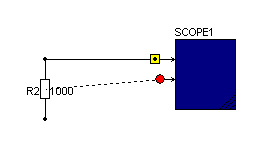Draw a wire from the output of the block SIGNAL to the first input of the scope and drag the third input of the scope over the capacitor.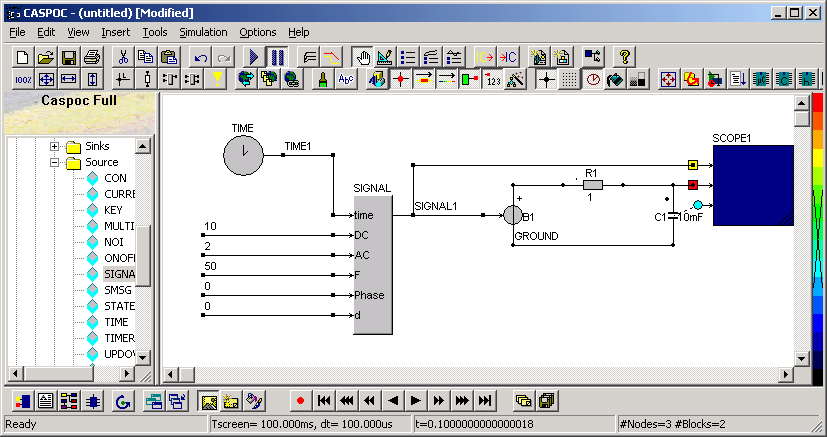Next we have to define the parameters for the simulation time. We have to set the total simulation time, the simulation step size and the numerical method. Open the simulation parameters dialog box by selecting Simulation/Simulation Parameters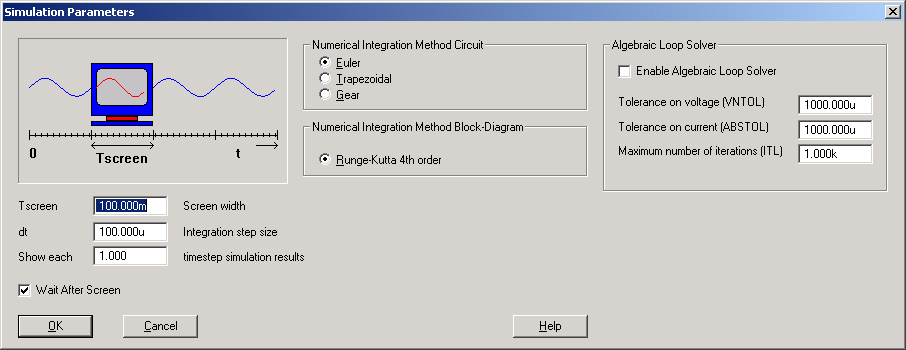The parameters that we have to set here are:
• TscreenThe total simulation time that will be simulated.
• dtThe step size. Each dt simulation results are displayed in the scope. Select dt small enough, for example, 100 to 1000 steps per period.
• Numerical integration method select the Euler numerical integration method for this example.
First we set the parameter Tscreen. Since our voltage source has a frequency of 50Hz, we select 5 periods to be simulated. The parameter Tscreen is thus set to 5*20ms = 100ms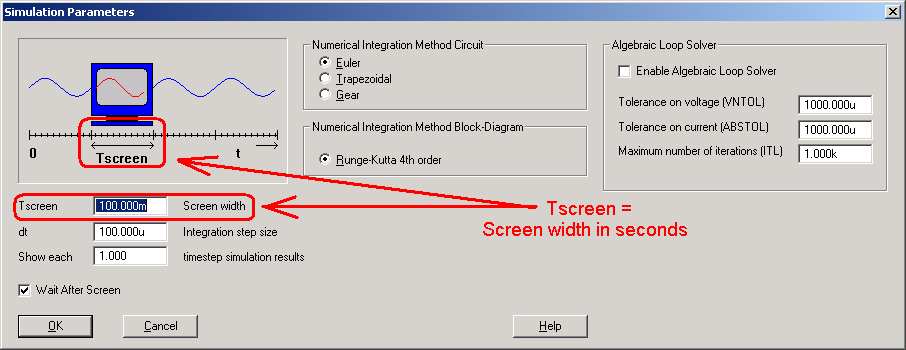Second we set the step size parameter dt for this simulation. The period of the voltage source is 20ms. We select a small step size of 100us for this simulation.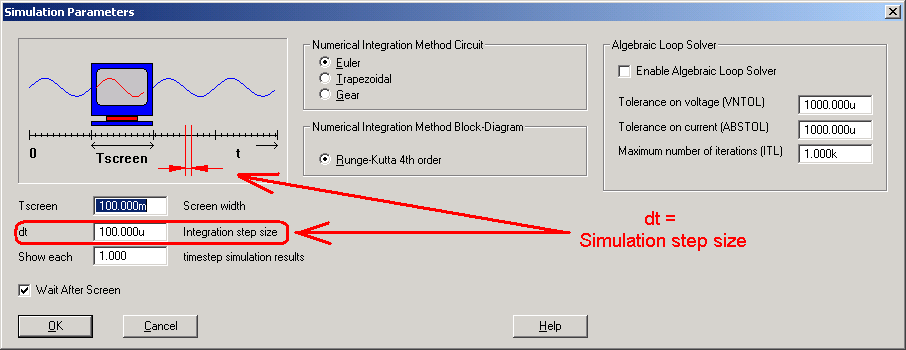The last parameter is the numerical method for the electrical circuit. Select Euler, which is a stabil method. The numerical method for the block diagram is default Runge Kutta 4th order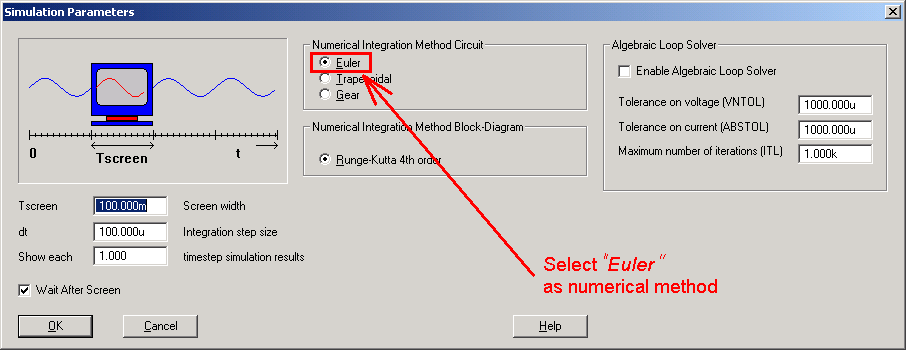Start the simulation by pressing the Play button or pressing the [Enter] key. The simulation is now started and the output from the block SIGNAL, output voltage and the current through the capacitor are shown in the scope. Open the scope by clicking it with the right mouse button.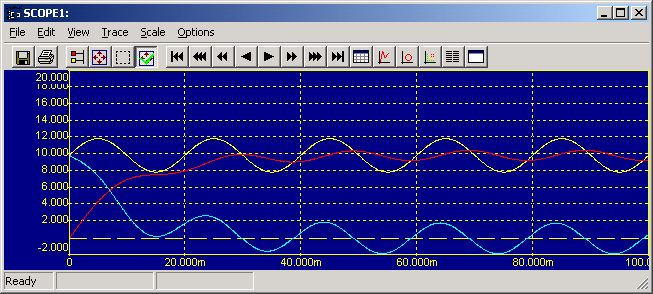The yellow trace is the output from the block SIGNAL. The red trace is the output voltage and the blue trace is the capacitor voltage. Compared to the original signal the ac value of the output voltage is damped by the capacitor of 10mF. If the capacitor value is decreased, the damping will be less and the output voltage will follow the sinusoidal wave form from the block SIGNAL. Open the properties dialog box for the capacitor by clicking it with the right mouse button and enter the value of 1mF at the field for the value of the capacitor.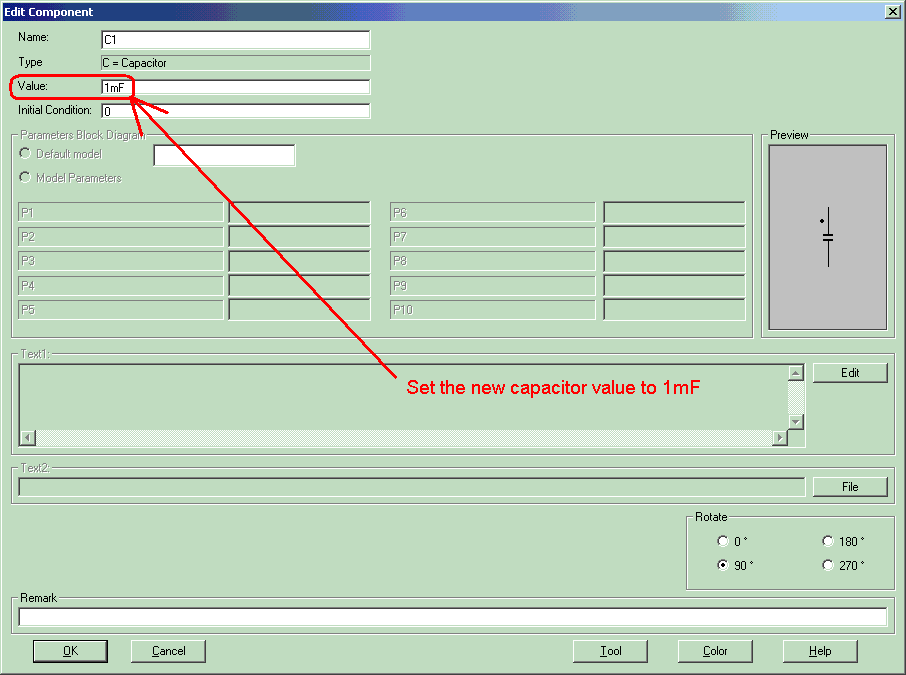Restart the simulation by pressing the Play button or continue the simulation by pressing the [Enter] key. The simulation is now started and the output from the block SIGNAL, the output voltage and the capacitor current are shown in the scope.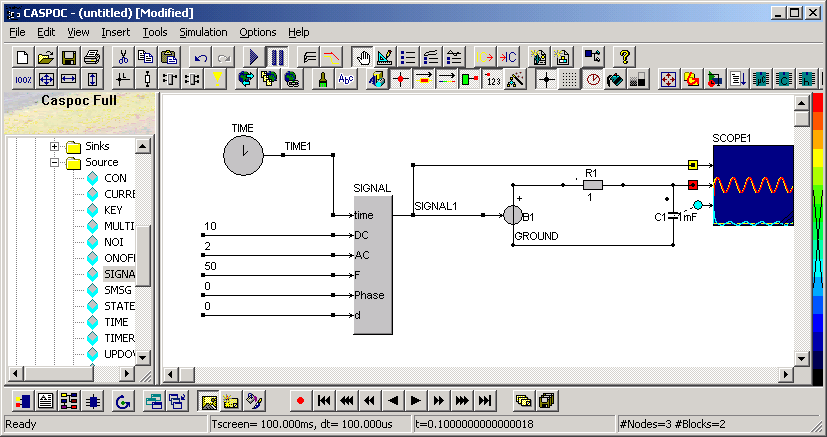Open the scope by clicking it with the right mouse button.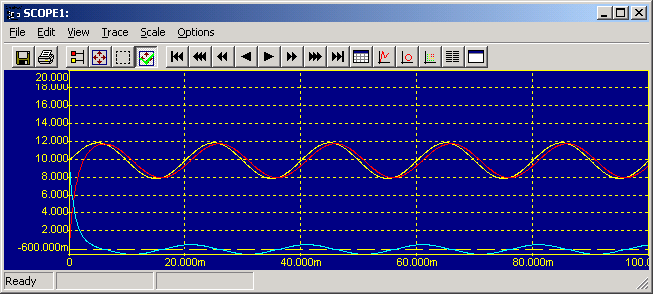The yellow trace is the output from the block SIGNAL. The red trace is the output voltage and the blue trace is the capacitor voltage.